Courses

# NCERT Exemplar (Part - 1) - Motion in a Straight Line Notes | EduRev

## NEET : NCERT Exemplar (Part - 1) - Motion in a Straight Line Notes | EduRev

The document NCERT Exemplar (Part - 1) - Motion in a Straight Line Notes | EduRev is a part of the NEET Course JEE Revision Notes.
All you need of NEET at this link: NEET

MULTIPLE CHOICE QUESTIONS

Q.1. Among the four graphs figure, there is only one graph for which average velocity over the time interval (0, T) can vanish for a suitably chosen T. Which one is it?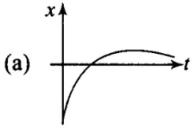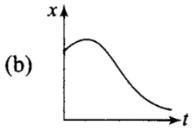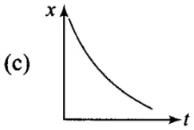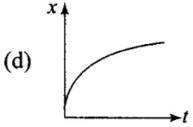Ans. (b)
Solution.

we need to identify the graph in which there is one displacement for different timings. it means that these displacements would be in opposite directions and when we add these opposite displacements, net displacement would be zero or average velocity would be zero. This thing is only possible in the graph(b).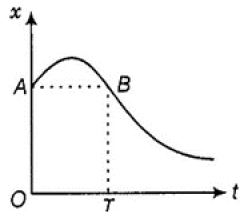If we draw a line parallel to time axis from the point (A) on the graph at t = 0 sec. This line Can intersect graph again at B.At this point, The change in displacement (O-T) time is zero i.e., displacement at A and B are equal so as the change in displacement is zero so the average velocity of body vanishes to zero.

Q.2. A lift is coming from 8th floor and is just about to reach 4th floor. Taking ground floor as origin and positive direction upwards for all quantities, which one of the following is correct?
(a) x < 0, v < 0, a > 0
(b) x > 0, v < 0, a < 0
(c) x > 0, v < 0, a > 0
(d) x > 0, v > 0, a < 0

Ans. (a)
Solution.
Key concept: The time rate of change of velocity of an object is called acceleration of the object.
It is a vector quantity. Its direction is same as that of change in velocity (Not of the velocity).
In the table: Possible ways of velocity change

 When only direction of velocity changes When only magnitude of velocity changes When both magnitude and direction of velocity change Acceleration perpendicular to velocity Acceleration parallel or anti­parallel to velocity Acceleration has two components—one is perpendicular to velocity and another parallel or anti­parallel to velocity E.g.: Uniform circular motion E.g.: Motion under gravity E.g: Projectile motion

Here we will take upward direction positive. As. the lift is coming in downward direction, the displacement will be negative. We have to see whether the motion is accelerating or retarding.
We know that due to downward motion displacement will be negative. When the lift reaches 4th floor and is about to stop velocity is decreasing with time, hence motion is retarding in nature. Thus, x < 0; a > 0. As displacement is in negative direction, velocity will also be negative, i.e. v < 0.
The motion of lift will be shown like this.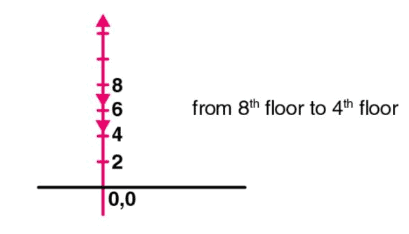Q.3. In one dimensional motion, instantaneous speed v satisfies 0 ≤ v < v0.
(a) The displacement in time T must always take non-negative values.
(b) The displacement x in time T satisfies – vo T < x < vo T.
(c) The acceleration is always a non-negative number.
(d) The motion has no turning points.

Ans. (b)
Solution.
Key concept: Instantaneous speed: It is the speed of a particle at a particular instant of time. When we say “speed”, it usually means instantaneous speed. The instantaneous speed is average speed for infinitesimally small time interval (i.e., ∆ → 0)
Thus, Instantaneous speed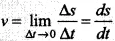As instantaneous speed is less than maximum speed. Then either the velocity is increasing or it is decreasing. For maximum and minimum displacement we have to keep in mind the magnitude and direction of maximum velocity.
As maximum velocity in positive direction is v0, magnitude of maximum velocity in opposite direction is also v0.
Maximum displacement in one direction = v0T Maximum displacement in opposite directions = -v0T Hence,-v0T<x< v0T.
Important point: We should not confuse with direction of velocities, i.e., in one direction it is taken as positive and in another direction it is taken as negative.

Q.4. A vehicle travels half the distance L with speed V1 and the other half with speed V2, then its average speed is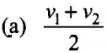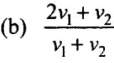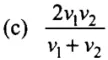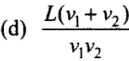Ans. (c)
Solution.
Time t1 taken in half distance = t1 = L/v1
Time t2 taken in half distance t2 = L/v2
Total time (t) taken in distance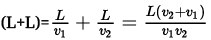Total distance = L+L = 2L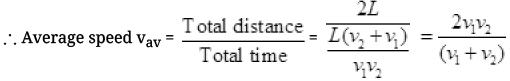Q.5. The displacement of a particle is given by x = (t – 2)2 where x is in metres and t in seconds. The distance covered by the particle in first 4 seconds is
(a) 4 m
(b) 8 m
(c) 12 m
(d) 16 m

Ans. (b)
Solution.
Key concept: Instantaneous velocity : Instantaneous velocity is defined as the rate of change of position vector of particles with time at a certain instant of time.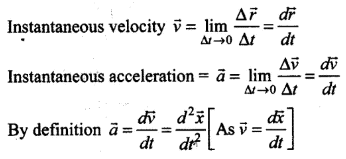i.e., if x  is given as a function of time, second time derivative of displacement gives acceleration.
In such type of problems we have to analyze whether the motion is accelerating or retarding. When acceleration is parallel to velocity, velocity of particle increases with time, i.e. motion is accelerated. And when acceleration is anti-parallel to velocity, velocity of particle decreases with time, i.e. motion is retarded. During retarding journey, particle will stop in between.
According to the problem, displacement of the particle is given as a function of time.
x = (t-2)2
By differentiating this equation w.r.t. time we get velocity of the particle as a function of time.
v = dx/ dt = d/dt (t-2)2 = 2(t – 2) m/s
If we again differentiate this equation w.r.t. time we will get acceleration of the particle as a function of time.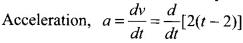= [1 - 0] = 2m/s2
When t = 0; v = -4 m/s
t = 2 s; v = 0 m/s
t = 4 s; v = 4 m/s
That means particle starts moving towards negative axis, then at t = 0, with a speed 4 m/s, at t = 2 it stops and start coming backward. At t = 4 its speed is +4 m/s.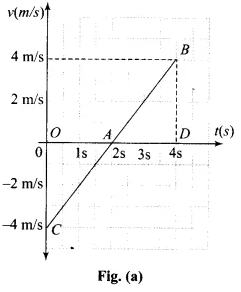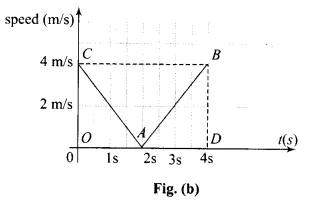v-t graph is shown in graph (a) and speed-time graph of the same situation is shown in graph (b).
Distance travelled = Area of the speed-time graph
= area OAC + area ABD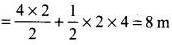Q.6. At a metro station, a girl walks up a stationary escalator in time t1. If she remains stationary on the escalator, then the escalator take her up in time t2. The time taken by her to walk up on the moving escalator will be
(a) (t1 + t2)/2
(b) t1t2/(t– t1)
(c) t1t2/(t+ t1)
(d) t1 – t2
Ans. (c)
Solution.
Let L be the length of the escalator.
Velocity of girl w.r.t. ground vg = L/t1
Velocity of escalator w.r.t. ground ve = L/t2
Effective Velocity of girl on moving escalator with respect to ground = vg + ve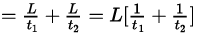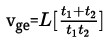∴ Time t taken by girl on moving escalator in going up the distance L is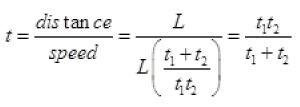Q.7. The variation of quantity A with quantity B, plotted in Fig. describes the motion of a particle in a straight line.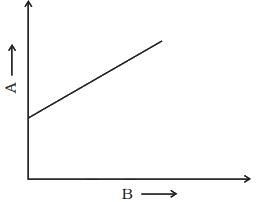(a) Quantity B may represent time.
(b) Quantity A is velocity if motion is uniform.
(c) Quantity A is displacement if motion is uniform.
(d) Quantity A is velocity if motion is uniformly accelerated.

Ans. (a, c, d)
Solution.
If B represents velocity then graph become the v-t graph is a straight line so it is uniformly accelerated motion, so motion is not uniform. Verifies option (a), (d).
If B represents time and A represents displacement, then graph become (s-t) graph. Here s-t graph is a straight line which represents uniform motion, so verifies the option (c).

Q.8. A graph of x versus t is shown in Fig. Choose correct alternatives from below.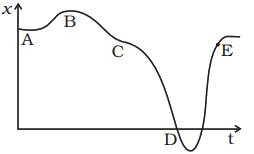(a) The particle was released from rest at t = 0.
(b) At B, the acceleration a > 0.
(c) At C, the velocity and the acceleration vanish.
(d) Average velocity for the motion between A and D is positive.
(e) The speed at D exceeds that at E.

Ans. (a, c, e)
Solution.

Slope of x-t graph gives v = dx/dt
At A graph (x-t) is parallel to the time axis, so dx/dt is zero or particle is at rest. After A, slope dx/dt increases, so velocity increases. Verifies option (a).
Tangent at B and C is a graph (x-t), that is parallel to the time axis, so dx/dt = 0 or v = 0.
It implies that acceleration a = 0 so it discards option (b) and verifies the option (c).
From graph the slope at D is greater than at E. So speed at D is greater than at E. Verifies the option (e).
Velocity at A is Zero as x-t parallel to time axis so average velocity at A is zero. At D displacement or slope is negative. So, the average velocity at D is negative not positive discards option(d).

Q.9. For the one-dimensional motion, described by x = t–sint
(a) x (t) > 0 for all t > 0.
(b) v (t) > 0 for all t > 0.
(c) a (t) > 0 for all t > 0.
(d) v (t) lies between 0 and 2

Ans. (a, d)
Solution.
Position of the particle is given as a function of time i.e. x = t – sint By differentiating this equation w.r.t. time we get velocity of the particle as a function of time.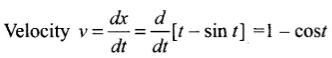If we again differentiate this equation w.r.t. time we will get acceleration of the particle as a function of time.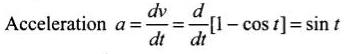As acceleration a > 0 for all t > 0
Hence, x(t) > 0 for all t > 0
Velocity v = 1 - cos
When, cos t = 1, velocity v = 0
vmax = 1 - (cos t)min = 1 - (- 1) = 2
vmin = 1 - (cos t)max = 1 - 1 = 0
Hence, v lies between 0 and 2.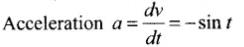When t = 0; x = 0, v = 0, a = 0
When t = π/2 ; x = positive, v = 0, a = -1 (negative)
When t = π, x = positive, v = positive, a = 0
When t = 2π; x = 0, v = 0, a = 0

Important points:
(i) When sinusoidal function is involved in an expression we should be careful about sine and cosine functions.
(ii) We should be very careful when calculating maximum and minimum value of velocity because it is in inverse relation with cost in the given expression.

Q.10. A spring with one end attached to a mass and the other to a rigid support is stretched and released.
(a) Magnitude of acceleration, when just released is maximum.
(b) Magnitude of acceleration, when at equilibrium position, is maximum.
(c) Speed is maximum when mass is at equilibrium position.
(d) Magnitude of displacement is always maximum whenever speed is minimum.
Ans.
(a, c)
Solution.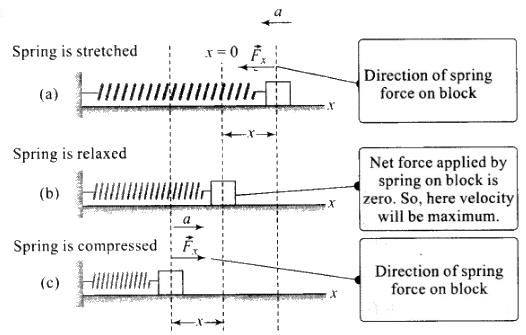As shown in the figure above when spring is stretched by length v, restoring force will be F = -kx (-ve sign shows that the force is always is the direction opposite to displacement x). Then the potential energy of the stretched spring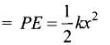The restoring force is central, hence when particle is released it will execute Simple Harmonic Motion about equilibrium position.
Acceleration will be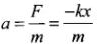At equilibrium position, x = 0 ⇒ a = 0
Hence, when just released. x = xmax
Hence, acceleration is maximum. Thus option (a) is correct.
At equilibrium whole PE will be converted to K.E. so KE will be maximum and hence, speed will be maximum. Thus option (c) is correct.

Q.11. A ball is bouncing elastically with a speed 1 m/s between walls of a railway compartment of size 10 m in a direction perpendicular to walls. The train is moving at a constant velocity of 10 m/s parallel to the direction of motion of the ball. As seen from the ground,
(a) The direction of motion of the ball changes every 10 seconds.
(b) Speed of ball changes every 10 seconds.
(c) Average speed of ball over any 20 second interval is fixed.
(d) The acceleration of ball is the same as from the train.

Ans. (b, c, d)
Solution.
In this problem, we have to observe the motion from different frames. Here the problem can be solved by the frame of the observer but here we must be clear that we are considering the motion from the ground so we just keep in mind the motion from frame of observer. Compared to the velocity of trains (10 m/s) speed of ball is less (1 m/s). (b, c, d) In this problem, we have to observe the motion from different frames. Here the problem can be solved by the frame of the observer but here we must be clear that we are considering the motion from the ground so we just keep in mind the motion from frame of observer. Compared to the velocity of trains (10 m/s) speed of ball is less (1 m/s).
The speed of the ball before collision with side of train is 10 + 1 = 11 m/s Speed after collision with side of train = 10 - 1 = 9 m/s As speed is changing after travelling 10 m and speed is 1 m/s, hence time duration of the changing speed is 10 s.
Since, the collision of the ball is perfectly elastic there is no dissipation of energy, hence total momentum and kinetic energy are conserved.
Since, the train is moving with a constant velocity, hence it will act as an inertial frame of reference as that of Earth and acceleration will be same in both frames.
Remember: We should not confuse with non-inertial and inertial frame of reference. A frame of reference that is not accelerating will be inertial.

Q.1. Match the following.

 Graph Characteristic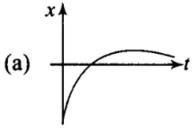(i) has v > 0 and a < 0 throughout.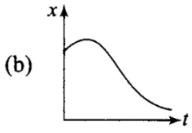(ii) has x > 0 throughout and has a point with v = 0 and a point with a = 0.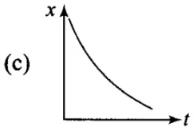(iii) has a point with zero displacement for t > 0.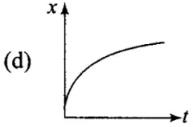(iv) has v < 0 and a > 0.

Ans. (a) (iii); (b) (ii); (c) (iv);(d) -(i)
Solution.

Let us pick graphs one by one.
In graph (a),
There is a point (B) on the curve for which displacement is zero. So curve, (a) matches with (iii).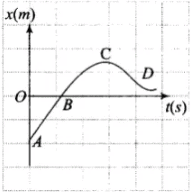In graph (b),
In this graph, x is positive (> 0) throughout and at point B the highest point of curve the slope of curve is zero. It means at this point v = dx/dt = 0 . Also at point C the dt curvature changes, it means at this point the acceleration of the particle should be zero or a = 0, So curve (b) matches with (ii).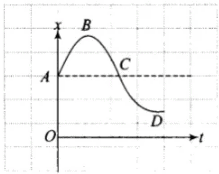In graph (c),
In this graph the slope is always negative, hence velocity will be negative or v < 0. Also x-t graph opens up, it represents positive acceleration. So curve (c) matches with (iv).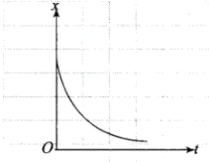In graph (d),
In this graph the slope is always positive, hence velocity will be positive or v > 0. Also x-t graph opens down, it represents negative acceleration. So curve (d) matches with (i).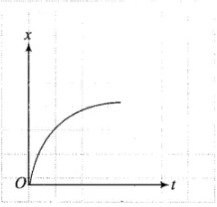Q.2. A uniformly moving cricket ball is turned back by hitting it with a bat for a very short time interval. Show the variation of its acceleration with time. (Take acceleration in the backward direction as positive).
Ans. Impulsive Force is generated by the bat: If we ignore the effect of gravity just by analyzing the motion of ball in horizontal direction only, then ball moving uniformly will return back with the same speed when a bat hits it.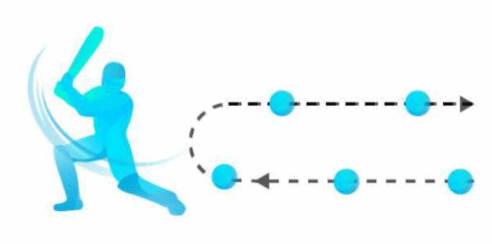Acceleration of the ball is zero just before it strikes the bat. When the ball strikes the bat, it gets accelerated due to the applied impulsive force by the bat.
The variation of acceleration with time is shown in the graph.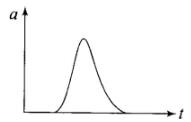Q.3. Give examples of a one-dimensional motion where
(a) The particle moving along positive x-direction comes to rest periodically and moves forward.
(b) The particle moving along positive x-direction comes to rest periodically and moves backward.

Ans. The equation which contains sine and cosine functions is periodic in nature.
(a) The particle will be moving along positive x-direction only if t > sin t We have displacement as a function of time, x(t) = t – sin t By differentiating this equation w.r.t. time we get velocity of the particle as a function of time.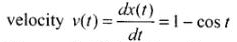If we again differentiate this equation w.r.t. time we will get acceleration of the particle as a function of time.
acceleration a(t) = dv/dt = sin t
when t = 0: x (t) = 0
when t = π;x (t) = π > 0
when t = 0; x (t) = 2π > 0
(b) Equation can be represented by
x(t) = sin t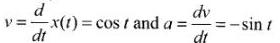At t = 0; x = 0, v = 1 (positive) and a = 0
At t = π/2 ; x = 1 (positive), v = 0 and a = -1 (negative)
At t = π; x = 0, v = -1 (negative) and a = 0
At t = 3π/2 ; x = -1 (negative), v = 0 and a = + 1 (positive)
At t = 2π, x = 0, v = 1 (positive) and a = 0
Hence the particle moving along positive x-direction comes to rest periodically and moves backward.
As displacement and velocity is involving sin t and cos t, hence these equations represent periodic nature.

Q.4. Give example of a motion where x > 0, v < 0, a > 0 at a particular instant.
Ans.
Let the motion is represented by
x(t) = A + Be-γt
Let A > B and y > 0     ...(i)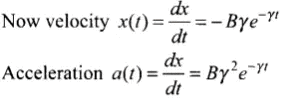Suppose we are considering any instant of time t, then from Eq. (i), we can say that
x(t) > 0; v (t) < 0 and a > 0

Q.5. An object falling through a fluid is observed to have acceleration given by a = g – bv where g = gravitational acceleration and b is constant. After a long time of release, it is observed to fall with constant speed. What must be the value of constant speed?
Ans. After long time of released the velocity becomes constant i.e.,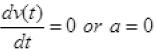Given acceleration is a=g-bv     ....(i)
0 = g - bv    [from (i)]
bv = g
v = g/b
Hence, the constant speed after a long time of release is (g/b).

Offer running on EduRev: Apply code STAYHOME200 to get INR 200 off on our premium plan EduRev Infinity!

233 docs

,

,

,

,

,

,

,

,

,

,

,

,

,

,

,

,

,

,

,

,

,

;